ORELA Math: Triangle Theorems & Proofs Chapter Exam

Exam Instructions:

Choose your answers to the questions and click 'Next' to see the next set of questions. You can skip questions if you would like and come back to them later with the yellow "Go To First Skipped Question" button. When you have completed the practice exam, a green submit button will appear. Click it to see your results. Good luck!

Page 1

Question 1 1. If triangle ACB is congruent to triangle YXZ, all of the following are true EXCEPT: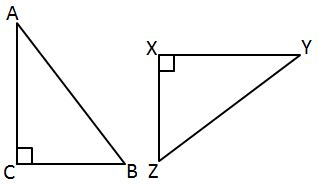Question 2 2. Triangle ABC is congruent to triangle XYZ. What reason can we use to explain why angle A is congruent to angle X?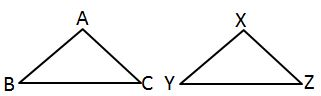Question 3 3. In the pictured triangle, AD is an angle bisector. What is the length of AC?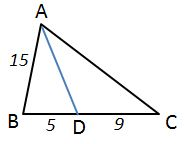Question 4 4. If triangle ABC is congruent to triangle ADC, what must also be true?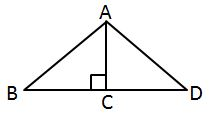Question 5 5. Which theorem can be used to establish congruence with the pictured triangles?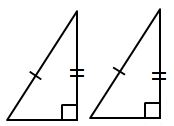Page 2

Question 7 7. In the pictured triangles, what reason can we use to explain that angle QPR is congruent to angle SPT?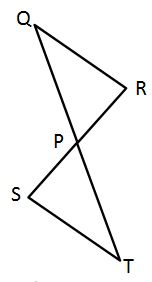Question 8 8. Find the measure of MN: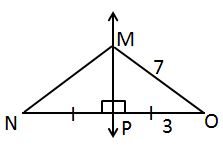Question 9 9. If the pictured triangles are congruent, what reason can be given?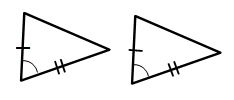Question 10 10. Which theorem or postulate can be used to establish congruence with the pictured triangles?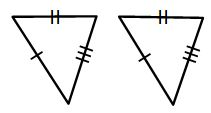Page 3

Question 11 11. All of the following statements about the pictured triangle must be true, EXCEPT: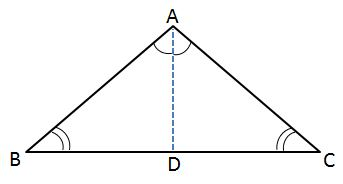Question 13 13. If triangle ABD is congruent to triangle CDB, CPCTC explains which of the following statements?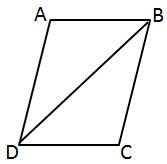Question 14 14. If triangle MNO is congruent to triangle PQR, CPCTC explains which of the following statements?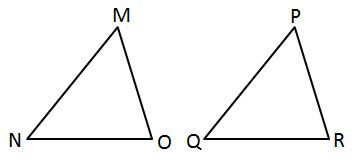Question 15 15. What additional information do you need to prove that triangle ABC is congruent to triangle DEF using the HA theorem?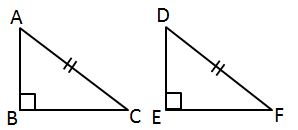Page 4

Question 18 18. Which theorem can be used to establish congruence with the pictured triangles?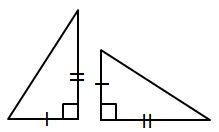Question 19 19. Which theorem or postulate can be used to establish congruence with the pictured triangles?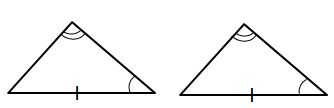Question 20 20. AD is a perpendicular bisector. Find the measure of AC: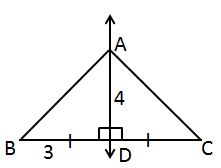Page 5

Question 21 21. Find the measure of XZ: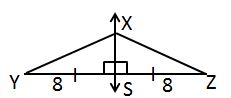Question 22 22. In the pictured triangle, XV is an angle bisector. What is the length of XY?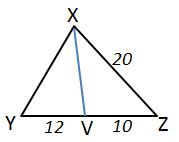Question 23 23. Which theorem can be used to establish congruence with the pictured triangles?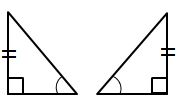Question 24 24. If triangle MNO is congruent to triangle STR, what must also be true?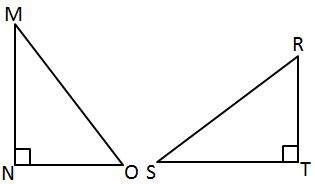Question 25 25. Which theorem or postulate can be used to establish congruence with the pictured triangles?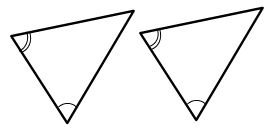Page 6

Question 27 27. What additional information do you need to prove that triangle ACD is congruent to triangle DBA using the HL theorem?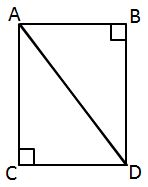Question 30 30. What additional information do you need to prove that triangle ABC is congruent to triangle DEF using the HL theorem?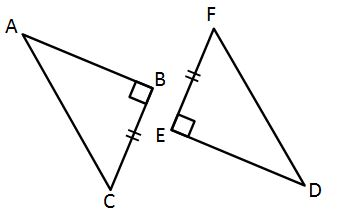ORELA Math: Triangle Theorems & Proofs Chapter Exam Instructions

Choose your answers to the questions and click 'Next' to see the next set of questions. You can skip questions if you would like and come back to them later with the yellow "Go To First Skipped Question" button. When you have completed the practice exam, a green submit button will appear. Click it to see your results. Good luck!

Support